Open In App
Related Articles
• Write an Interview Experience
• R Language Tutorial

# Data visualization with R and ggplot2

Data visualization with R and ggplot2ggplot2 package in R Programming Language also termed as Grammar of Graphics is a free, open-source, and easy-to-use visualization package widely used in R. It is the most powerful visualization package written by Hadley Wickham.

It includes several layers on which it is governed. The layers are as follows:

## Building Blocks of layers with the grammar of graphics

• Data: The element is the data set itself
• Aesthetics: The data is to map onto the Aesthetics attributes such as x-axis, y-axis, color, fill, size, labels, alpha, shape, line width, line type
• Geometrics: How our data being displayed using point, line, histogram, bar, boxplot
• Facets: It displays the subset of the data using Columns and rows
• Statistics: Binning, smoothing, descriptive, intermediate
• Coordinates: the space between data and display using Cartesian, fixed, polar, limits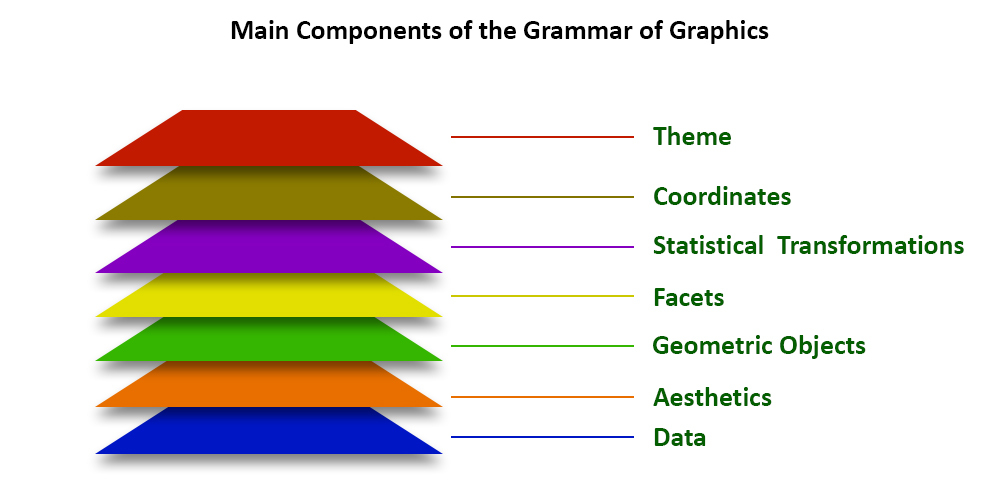## Dataset Used

mtcars(motor trend car road test) comprise fuel consumption and 10 aspects of automobile design and performance for 32 automobiles and come pre-installed with dplyr package in R.

## R

 `# Installing the package``install.packages``(``"dplyr"``)` `# Loading package``library``(dplyr)` `# Summary of dataset in package``summary``(mtcars)`

Output:

```      mpg             cyl             disp             hp
Min.   :10.40   Min.   :4.000   Min.   : 71.1   Min.   : 52.0
1st Qu.:15.43   1st Qu.:4.000   1st Qu.:120.8   1st Qu.: 96.5
Median :19.20   Median :6.000   Median :196.3   Median :123.0
Mean   :20.09   Mean   :6.188   Mean   :230.7   Mean   :146.7
3rd Qu.:22.80   3rd Qu.:8.000   3rd Qu.:326.0   3rd Qu.:180.0
Max.   :33.90   Max.   :8.000   Max.   :472.0   Max.   :335.0
drat             wt             qsec             vs
Min.   :2.760   Min.   :1.513   Min.   :14.50   Min.   :0.0000
1st Qu.:3.080   1st Qu.:2.581   1st Qu.:16.89   1st Qu.:0.0000
Median :3.695   Median :3.325   Median :17.71   Median :0.0000
Mean   :3.597   Mean   :3.217   Mean   :17.85   Mean   :0.4375
3rd Qu.:3.920   3rd Qu.:3.610   3rd Qu.:18.90   3rd Qu.:1.0000
Max.   :4.930   Max.   :5.424   Max.   :22.90   Max.   :1.0000
am              gear            carb
Min.   :0.0000   Min.   :3.000   Min.   :1.000
1st Qu.:0.0000   1st Qu.:3.000   1st Qu.:2.000
Median :0.0000   Median :4.000   Median :2.000
Mean   :0.4062   Mean   :3.688   Mean   :2.812
3rd Qu.:1.0000   3rd Qu.:4.000   3rd Qu.:4.000
Max.   :1.0000   Max.   :5.000   Max.   :8.000  ```

## Example of ggplot2 package in R Programming

We devise visualizations on mtcars dataset which includes 32 car brands and 11 attributes using ggplot2 layers.

### Data Layer:

In the data Layer we define the source of the information to be visualize, let’s use the mtcars dataset in the ggplot2 package

## R

 `library``(ggplot2)``library``(dplyr)` `ggplot``(data = mtcars) +``  ``labs``(title = ``"MTCars Data Plot"``)`

Output: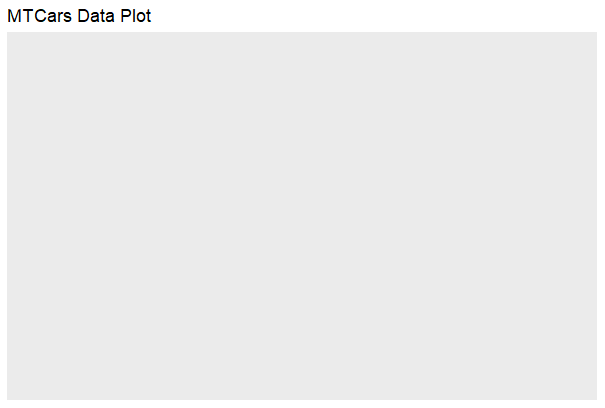Data visualization with R and ggplot2

### Aesthetic Layer:

Here we will display and map dataset into certain aesthetics.

## R

 `# Aesthetic Layer``ggplot``(data = mtcars, ``aes``(x = hp, y = mpg, col = disp))+`` ``labs``(title = ``"MTCars Data Plot"``)`

Output: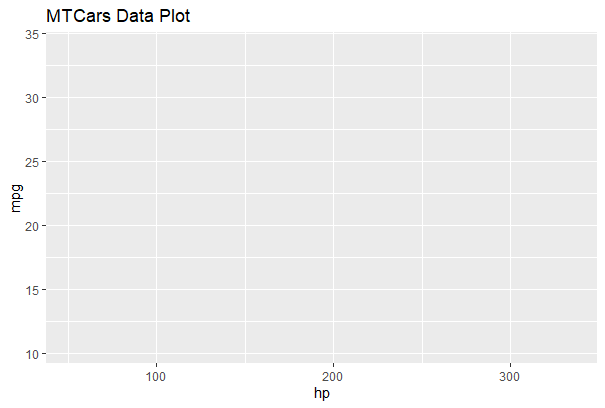Data visualization with R and ggplot2

### Geometric layer:

In geometric layer control the essential elements, see how our data being displayed using point, line, histogram, bar, boxplot

## R

 `# Geometric layer``ggplot``(data = mtcars, ``aes``(x = hp, y = mpg, col = disp)) +``  ``geom_point``() +``  ``labs``(title = ``"Miles per Gallon vs Horsepower"``,``       ``x = ``"Horsepower"``,``       ``y = ``"Miles per Gallon"``)`

Output: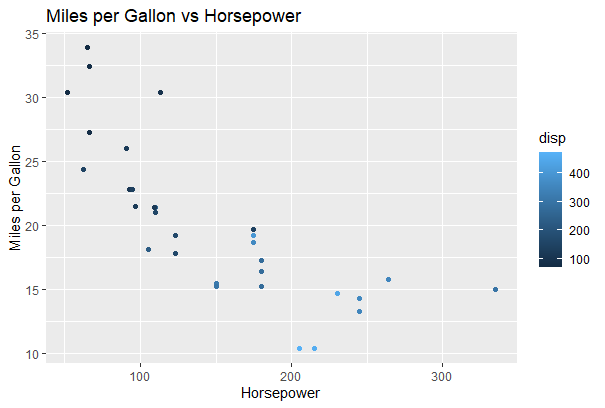Data visualization with R and ggplot2

Geometric layer: Adding Size, color, and shape and then plotting the Histogram plot

## R

 `# Adding size``ggplot``(data = mtcars, ``aes``(x = hp, y = mpg, size = disp)) +``  ``geom_point``() +``  ``labs``(title = ``"Miles per Gallon vs Horsepower"``,``       ``x = ``"Horsepower"``,``       ``y = ``"Miles per Gallon"``)` `# Adding shape and color``ggplot``(data = mtcars, ``aes``(x = hp, y = mpg, col = ``factor``(cyl),``       ``shape = ``factor``(am))) +``geom_point``() +``  ``labs``(title = ``"Miles per Gallon vs Horsepower"``,``       ``x = ``"Horsepower"``,``       ``y = ``"Miles per Gallon"``)` `# Histogram plot``ggplot``(data = mtcars, ``aes``(x = hp)) +``  ``geom_histogram``(binwidth = 5) +``  ``labs``(title = ``"Histogram of Horsepower"``,``       ``x = ``"Horsepower"``,``       ``y = ``"Count"``)`

Output: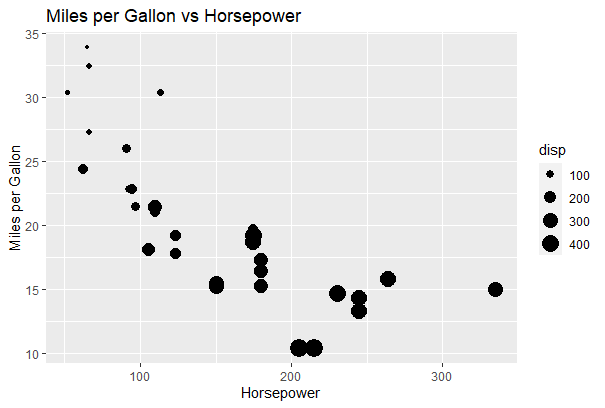Data visualization with R and ggplot2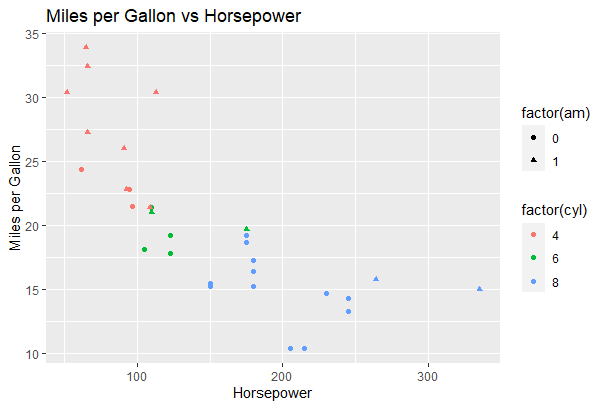Data visualization with R and ggplot2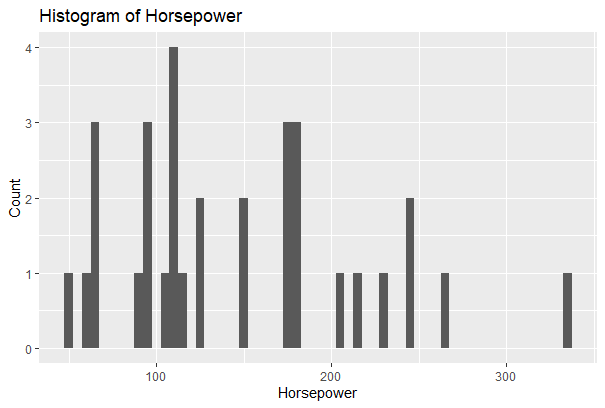Data visualization with R and ggplot2

### Facet Layer:

It is used to split the data up into subsets of the entire dataset and it allows the subsets to be visualized on the same plot. Here we separate rows according to transmission type and Separate columns according to cylinders

## R

 `# Facet Layer``# Separate rows according to transmission type``p <- ``ggplot``(data = mtcars, ``aes``(x = hp, y = mpg, shape = ``factor``(cyl))) + ``geom_point``()` `p + ``facet_grid``(am ~ .) +``  ``labs``(title = ``"Miles per Gallon vs Horsepower"``,``       ``x = ``"Horsepower"``,``       ``y = ``"Miles per Gallon"``)` `# Separate columns according to cylinders``p <- ``ggplot``(data = mtcars, ``aes``(x = hp, y = mpg, shape = ``factor``(cyl))) + ``geom_point``()` `p + ``facet_grid``(. ~ cyl) +``  ``labs``(title = ``"Miles per Gallon vs Horsepower"``,``       ``x = ``"Horsepower"``,``       ``y = ``"Miles per Gallon"``)`

Output: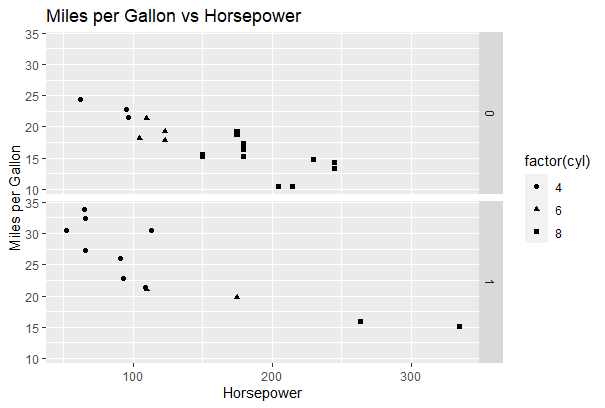Data visualization with R and ggplot2Data visualization with R and ggplot2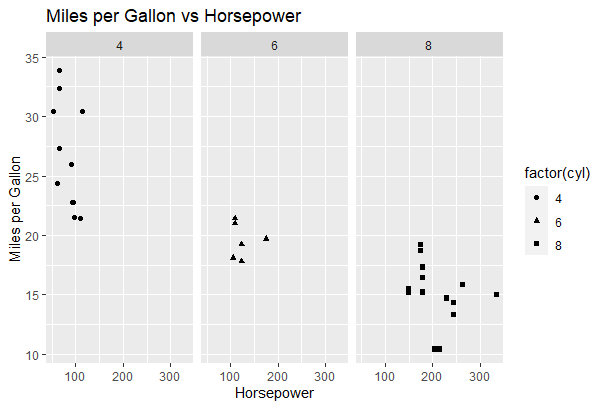Data visualization with R and ggplot2

### Statistics layer

In this layer, we transform our data using binning, smoothing, descriptive, intermediate

## R

 `ggplot``(data = mtcars, ``aes``(x = hp, y = mpg)) +``  ``geom_point``() +``  ``stat_smooth``(method = lm, col = ``"red"``) +``  ``labs``(title = ``"Miles per Gallon vs Horsepower"``)`

Output: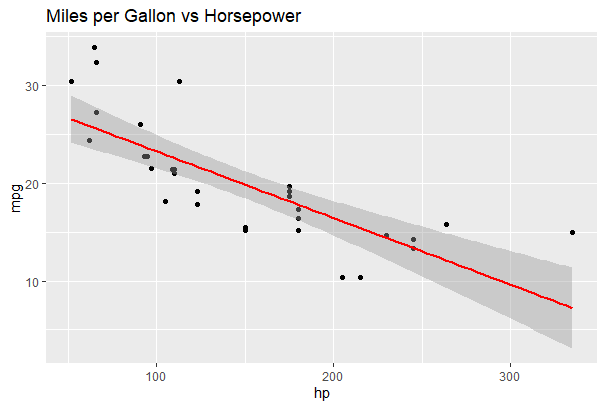Data visualization with R and ggplot2

### Coordinates layer:

In these layers, data coordinates are mapped together to the mentioned plane of the graphic and we adjust the axis and changes the spacing of displayed data with Control plot dimensions.

## R

 `ggplot``(data = mtcars, ``aes``(x = wt, y = mpg)) +``  ``geom_point``() +``  ``stat_smooth``(method = lm, col = ``"red"``) +``  ``scale_y_continuous``(``"Miles per Gallon"``, limits = ``c``(2, 35), expand = ``c``(0, 0)) +``  ``scale_x_continuous``(``"Weight"``, limits = ``c``(0, 25), expand = ``c``(0, 0)) +``  ``coord_equal``() +``  ``labs``(title = ``"Miles per Gallon vs Weight"``,``       ``x = ``"Weight"``,``       ``y = ``"Miles per Gallon"``)`

Output: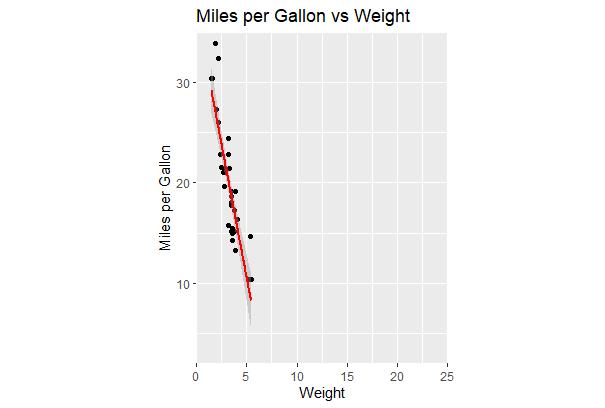Data visualization with R and ggplot2

Coord_cartesian() to proper zoom in:

## R

 `# Add coord_cartesian() to proper zoom in``ggplot``(data = mtcars, ``aes``(x = wt, y = hp, col = am)) +``                        ``geom_point``() + ``geom_smooth``() +``                        ``coord_cartesian``(xlim = ``c``(3, 6))`

Output: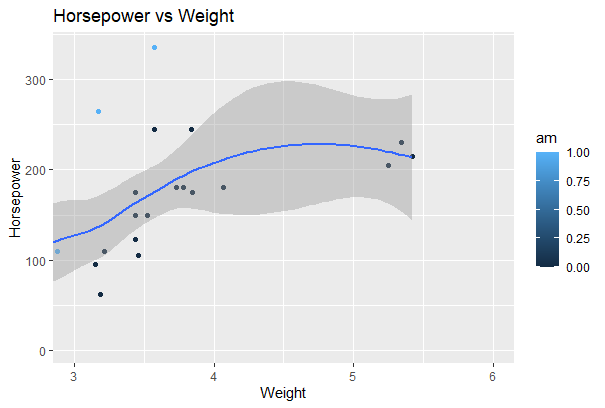Data visualization with R and ggplot2

### Theme Layer:

This layer controls the finer points of display like the font size and background color properties.

Example 1: Theme layer – element_rect() function

## R

 `ggplot``(data = mtcars, ``aes``(x = hp, y = mpg)) +``  ``geom_point``() +``  ``facet_grid``(. ~ cyl) +``  ``theme``(plot.background = ``element_rect``(fill = ``"blue"``, colour = ``"gray"``)) +``  ``labs``(title = ``"Miles per Gallon vs Horsepower"``)`

Output: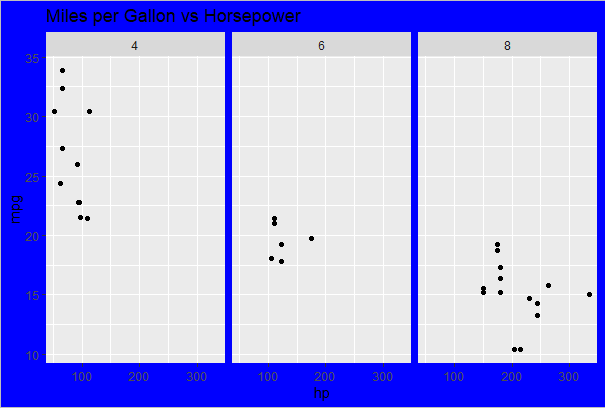Data visualization with R and ggplot2

Example 2:

## R

 `ggplot``(data = mtcars, ``aes``(x = hp, y = mpg)) +``        ``geom_point``() + ``facet_grid``(am ~ cyl) +``        ``theme_gray``()+`` ``labs``(title = ``"Miles per Gallon vs Horsepower"``)`

Output: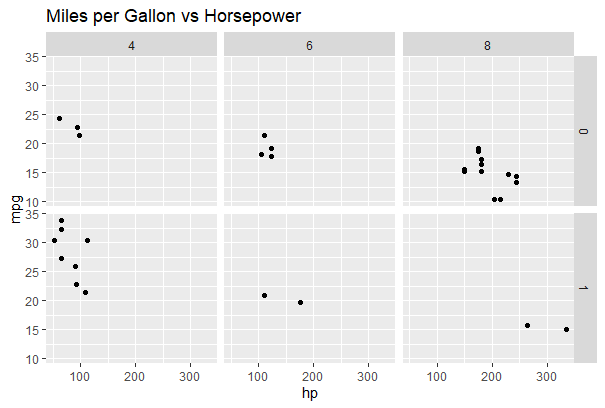Data visualization with R and ggplot2

ggplot2 provides various types of visualizations. More parameters can be used included in the package as the package gives greater control over the visualizations of data. Many packages can integrate with the ggplot2 package to make the visualizations interactive and animated.

### Save and extract R plots:

To save and extract plots in R, you can use the ggsave function from the ggplot2 package. Here’s an example of how to save and extract plots:

## R

 `# Create a plot``plot <- ``ggplot``(data = mtcars, ``aes``(x = hp, y = mpg)) +``  ``geom_point``() +``  ``labs``(title = ``"Miles per Gallon vs Horsepower"``)` `# Save the plot as an image file (e.g., PNG)``ggsave``(``"plot.png"``, plot)` `# Save the plot as a PDF file``ggsave``(``"plot.pdf"``, plot)` `# Extract the plot as a variable for further use``extracted_plot <- plot``plot`

Output: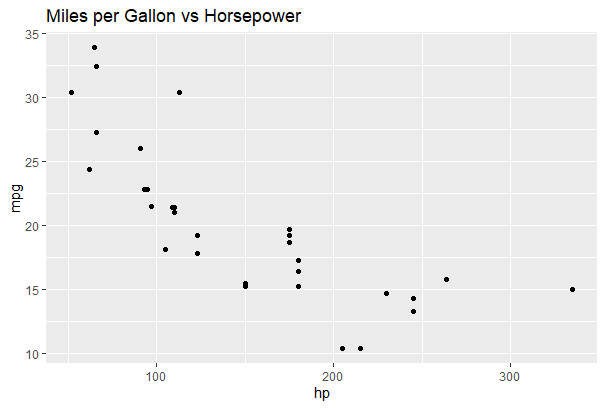Data visualization with R and ggplot2

In this demonstration, I used ggplot to construct a plot and the ggsave function to save it as a PDF file (plot.pdf) and a PNG image file (plot.png). By including the correct file extension, you can indicate the intended file format.

You may easily give the ggplot object to a variable, as demonstrated with extracted_plot, to extract the plot as a variable for later usage.

Be sure to substitute your unique plot and desired file names for the plot code and file names (plot.png and plot.pdf).